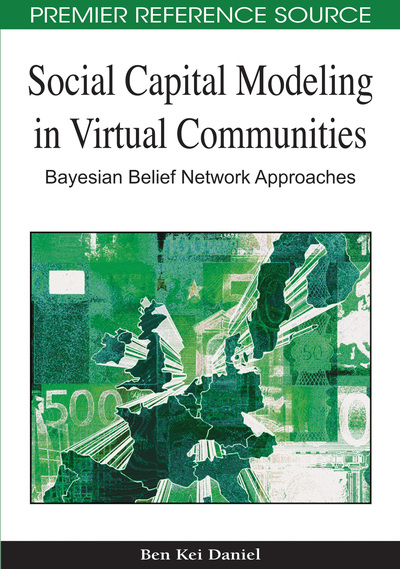Sensitivity Analysis

DOI: 10.4018/978-1-60566-663-1.ch013
Available
\$37.50
No Current Special Offers

Abstract

Sensitivity analysis is a mathematical technique for investigating the effects of inaccuracies in the parameters of a mathematical model. It analyses how variation in the output of a model (numerical or otherwise) can be apportioned qualitatively or quantitatively to different sources of data. Sensitivity analysis is an important statistical validation technique in Bayesian Modelling. It is used to ascertain how a given model output depends upon or determines its input parameters. It often carried out to ensure the quality and accuracy of a model and a way of checking the robustness and reliability of assumptions built into a model. This Chapter offers an accessible introduction to sensitivity analysis of Bayesian models. The Chapter should have been a section in Chapter 12 and presented before the scenarios sections in Chapter 12. But because sensitivity analysis itself is a complex subject, it was deemed wise to present it as a complete Chapter on its own.
Chapter Preview
Top

Overview Of Sensitivity Analysis

Modellers from a wide range of disciplines, including economics, educational assessment and evaluation and engineering have immensely benefited from the employment of sensitivity analysis techniques to valid their models. In a broad-spectrum sensitivity analysis within the Bayesian statistical approaches helps modellers to determine the spread of probability distribution of a particular variable or set of variables (parameters) and how they influences other variables or are influenced by values of other variables within the model. In other words, the purpose of sensitivity analysis is to know how sensitive a variable’s value is to the other variables in the model. If it is very sensitive, we may want to know the state of that variable, and then invest more effort in determining the values of all the variables that substantially influences it.

Parameter sensitivity, an example of sensitivity analysis involves setting up a series of tests in where a modeller sets different parameter values to see how a change in one parameter in the model causes a change in the behaviour of one or more variables within the model. By showing how the model behaviour responds to changes in parameter values, sensitivity analysis serves as a useful tool during the process of building a model as well as in the evaluation of the model. Since modelling can be based on a lot of assumptions, some which might be less accurate, sensitivity analysis helps modellers to build confidence in the model by studying the uncertainties that are often associated with parameters in models.

Further, since some parameters in Bayesian models might represent probability values that are inaccurate, Sensitivity analysis is conducted to rectify this inaccuracy.

Sensitivity analysis allows model builders to determine what level of accuracy is needed for a parameter to make the model sufficiently useful and valid. If tests reveal that the model is insensitive, then it may be possible to use statistical estimation techniques to achieve greater precision. Within a Bayesian models, sensitivity analysis can also reveal the parameters that are reasonable or sensitive to use in the model. In practice, it might not be obvious to identify sensitive parameters but experimenting with a wide range of values can offer insights into behaviour of a model in extreme situations.

A Bayesian model is defined by a series of variables and these are treated as input parameters aimed at representing and understanding the behaviour of a system being modelled. Bayesian variables together with the assumptions made during the modelling process are often subject to many sources of uncertainty including errors of measurement, absence of information and poor or partial understanding of the driving forces and mechanisms. Such range of uncertainties can impose limitation and sometimes undermine the model’s output.

Good modelling practice requires that the model builder provide an evaluation of the confidence in the model, possibly assessing the uncertainties associated with the modeling process and with the outcome of the model itself. In models involving many input variables sensitivity analysis is an essential ingredient of model building and quality assurance. It ensures that input knowledge gathered from experts is accurate and that logic used for building the structure of the model and the assumptions underlying it are valid as well.

Complete Chapter List

Search this Book:
Reset